机器学习-数据科学库（HM）_第3节_numpy1389-李同学

shush juzhen list

numpy

什么是numpy

-为什么要学习numpy?
– 快速
– 方便
– 科学计算的基础库

• 什么是numpy?
• 一个在Python中做科学计算的基础库，重在数值计算，也是大部分PYTHON科学计算库的基础库，多用于在大型、多维数组上执行数值运算。
• 数组：矩阵、array、list

numpy基础

numpy创建数组(矩阵)

• 创建数组：

import numpy as np
t1 = np.array([1,2,3,])
t2 = np.array(range(10))
t3 = np.arange(4,10,2)

• 数组的类名：

type(a)
> numpy.ndarray

数据类型的操作

• 数据的类型：

a.dtype
> dtype(“int64”)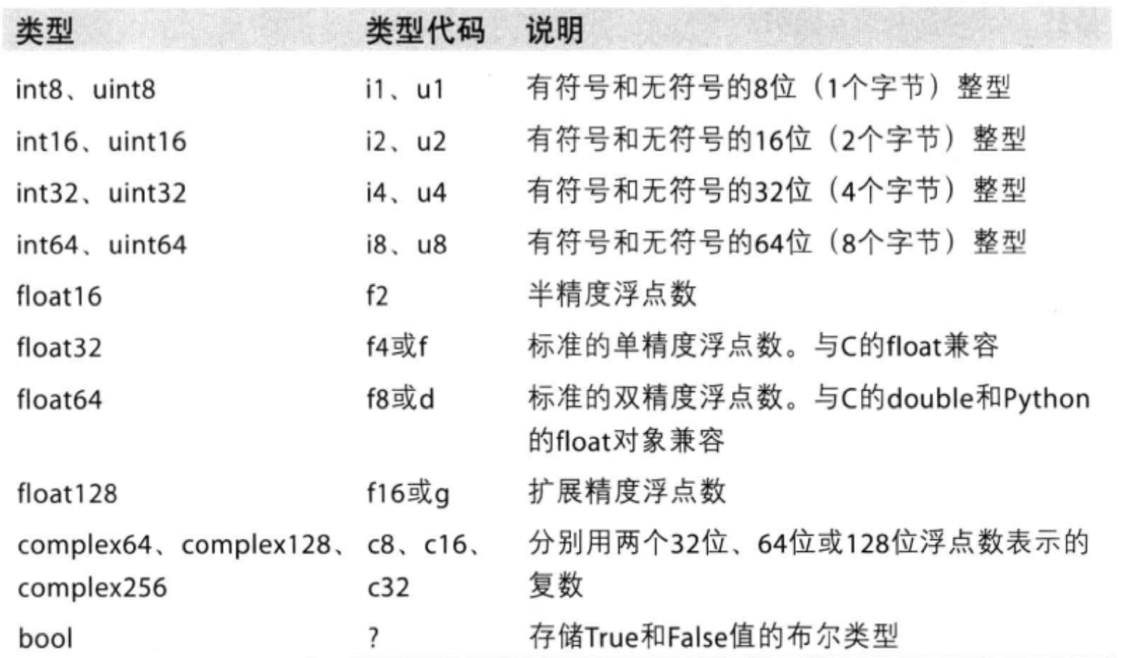• 制定创建的数组的数据类型：

t5 = np.array([1,1,0,1,0,0], dtype=bool)
t5 = np.array([1,1,0,1,0,0], dtype="?")

• 修改数组的数据类型：

t6 = t5.astype(“int8”)

• 修改浮点型的小数位数：

• t8 = np.round(t7,2) # 保留2小数点后两位
• round(random.random(), 3)
• “%.2f”%random.random() # 随机生成一个小数点后保留2为的float

数组的形状

a = np.array([[3,4,5,6,7,8], [4,5,6,7,8,9]])

• 查看数组的形状：

a.shape
>(2, 6)  # 最里面的列表有6个元素，外层列表有2个元素；2行6列
t3 = np.array([[[1,2,3], [4,5,6]]
[[7,8,9], [10,11,12]]])
t3.shape
>(2,2,3)	# 2块，每块2行3列

• 修改数组的形状，不会对原来数组进行修改

b = a.reshape((3,4))
>array([[3,4,5,6],
[7,8,4,5]
[6,7,8,9]])

# 把数组转化为一维度数据：方法一
b.reshape((12,))
array([3,4,5,6,7,8,4,5,6,7,8,9])

# 把数组转化为一维度数据：方法二，数组的维度未知
b.flattern()
array([3,4,5,6,7,8,4,5,6,7,8,9])

# 把数组转化为一维度数据：方法三，数组的维度未知
b.reshape((b.shape*b.shape,))

# 两种转化为一维度的错误辨析
b.reshape((1,12))
array([[3,4,5,6,7,8,4,5,6,7,8,9]])

b.reshape((12,1))
array([,
,
,
....
,
])
b.reshape((1,24))

数组和数的计算

• a+1
• a*3
• numpy中的广播机制使在运算过程中，加减乘除的运算被广播到所有的元素上面。
• a+b / a*b ：
• 当两个数组形状一样时，二者进行运算，对应位进行加减乘除计算• 当两个数组形状不一样，但是a为单行/列与b的行/列个数一样时， 则可以在每一行/列上单独与a进行计算。广播原则

• 如果两个数组的后缘维度（trailing dimension，即从末尾开始算起的维度）的轴长度享福或其中一方的长度为1，则认为他们是广播兼容的。广播会在缺失和（或）长度为1的维度上进行。
• 可以把维度理解为shape所对应的数字个数
• shape为(3,3,3)的数组不能和(3,2)的数组进行计算
• shape为(3,3,2)的数组能够和(3,2)的数组进行计算
• shape为(3,3,2)的数组能够和(3,3)的数组进行计算

轴

• 在numpy中可以理解为方向，使用0, 1, 2…数字表示，对于一个一维数组，只有一个0轴,对于2维数组(shape(2, 2))，有0(行)轴和1(列)轴，对于三维数组(shape(2, 2, 3))，有0(块), 1(行), 2(列)轴。
• 有了轴的概念之后,我们计算会更加方便，比如计算一个2维数组的平均值，必须指定是计算哪个方向上面的数字的平均值。

二维数组的轴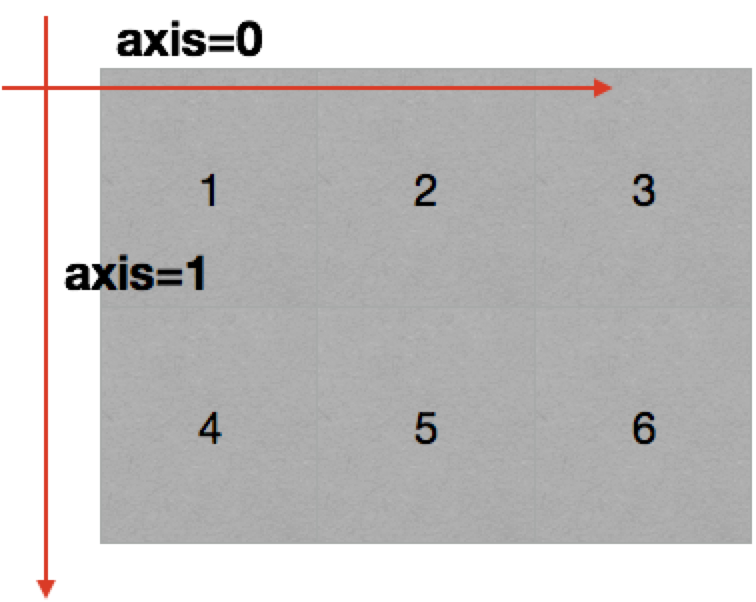三维数组的轴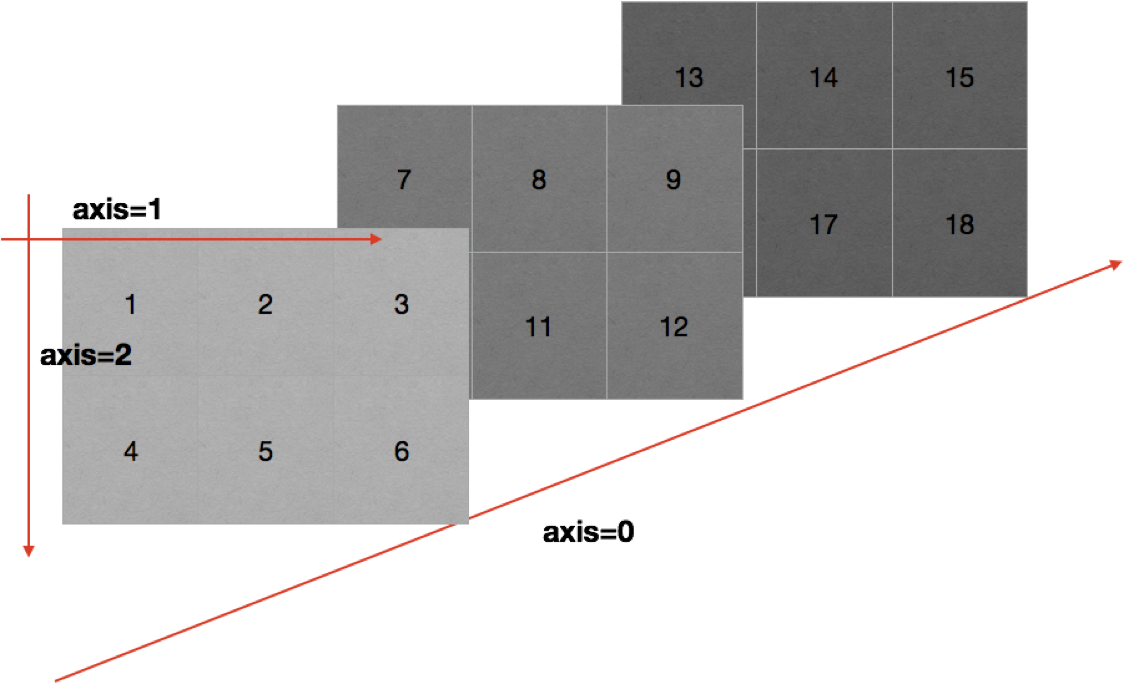numpy常用方法

numpy读取数据

• numpy是从CSV（Comma-Separated Value）逗号分隔值文件中读取数据的，一般不用，常用pandas。
• delimiter：指定边界符号，不指定会导致每行数据为一个整体的字符串，从而报错
• dtype：默认情况下对于较大的数据会将其变为科学计数法。
• unpack的效果和transpose一样，列行互换。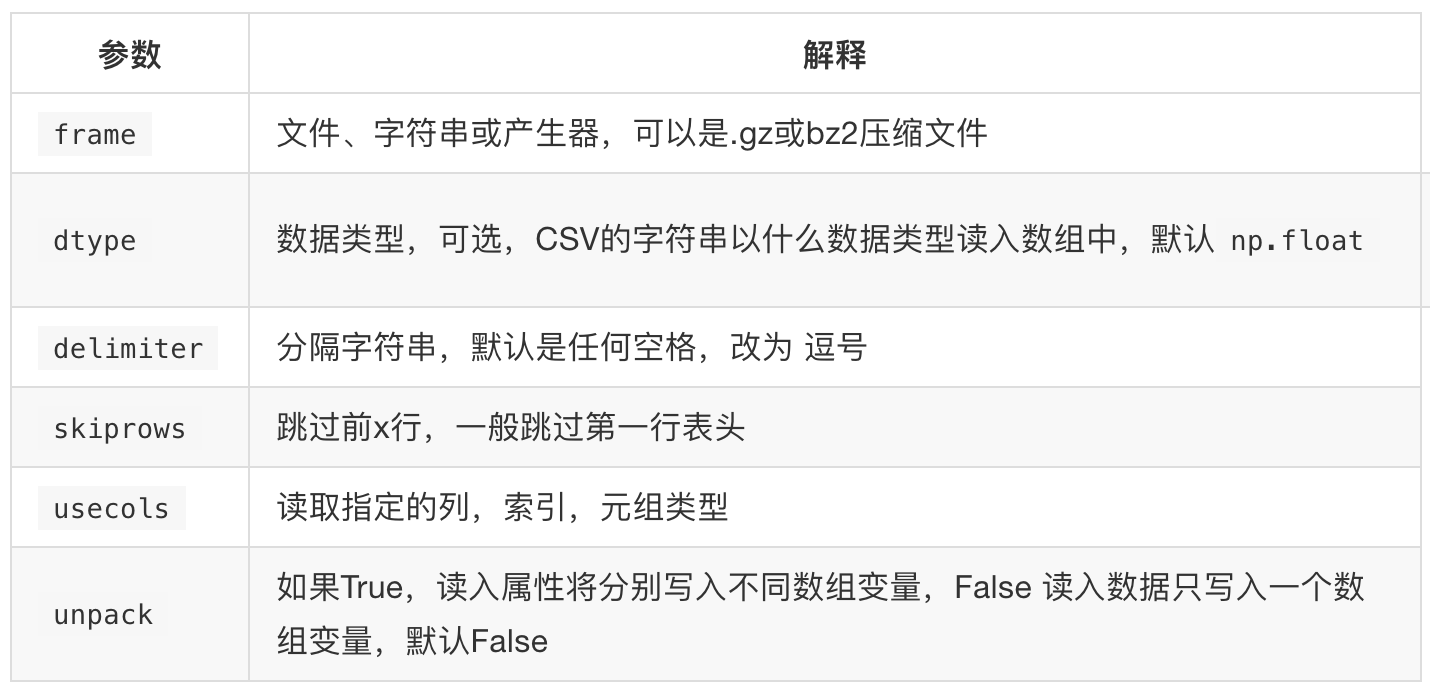numpy读取和储存数据的实现

# coding:utf-8
import numpy as np

t = np.loadtxt(us_file_path, delimiter=",", dype="int", unpack=True)

numpy中的转置（transpose）

• 转置是一种变换，对于numpy中的数组来说，就是在对角线方向交换数据，目的也是为了更方便的去处理数据。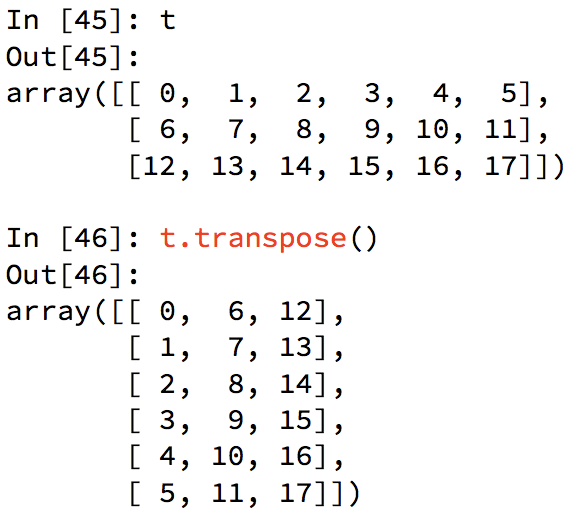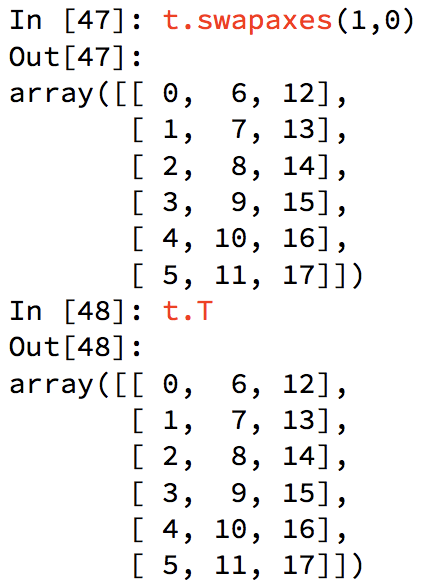numpy索引和切片

• 取行(np.array)：t
• 取连续的多行：t[2:]
• 取不连续的多行：t[[2, 8, 18]]
• 取行/列： t[行, 列]
• 取第二行：t[1, :]
• 取列：t[:, 0]
• 取连续的多列：t[:, 2:]
• 取不连续的多列：t[:, [0, 2]]
• 取第3行、第4列的值(np.int64)：t[3, 4]
• 取多行和多列：取交叉点的array
• 取第3行到第5行，第2列到第4列：t[2:5, 1:4]
• 取多个不相邻的点：t[[0, 2], [0, 1]]
• 选出来的结果是(0, 0)，(2, 1)numpy中数值的修改

• 直接对索引进行赋值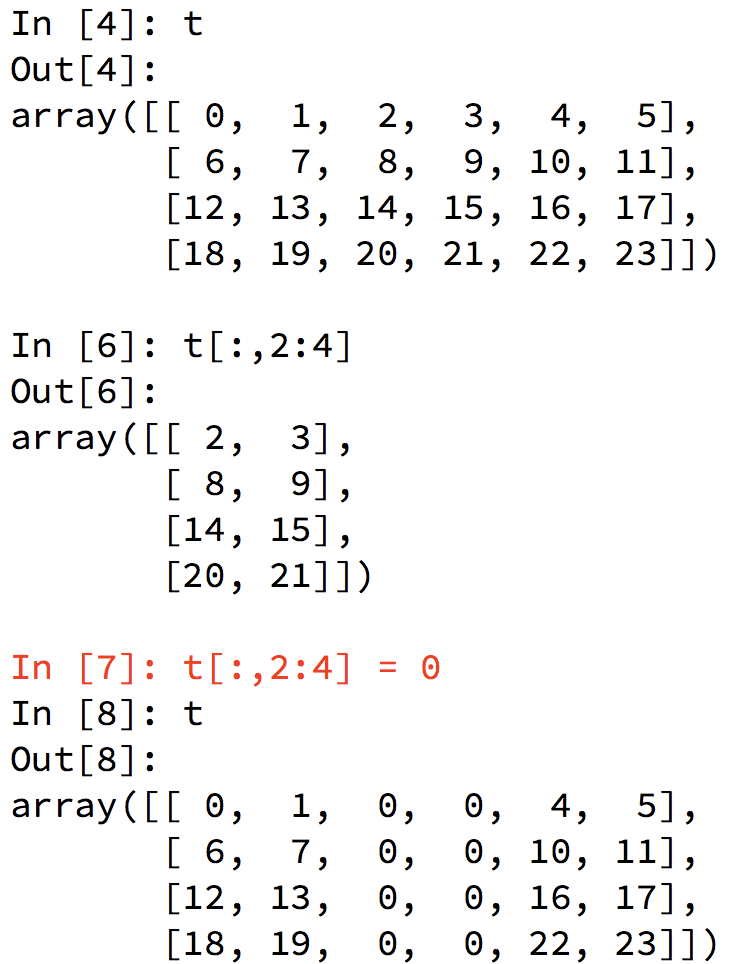numpy中的布尔索引

• 把t2中小于10的数字替换成3numpy中三元运算符

• 把t中小于10的数字替换成0，大于10的替换成10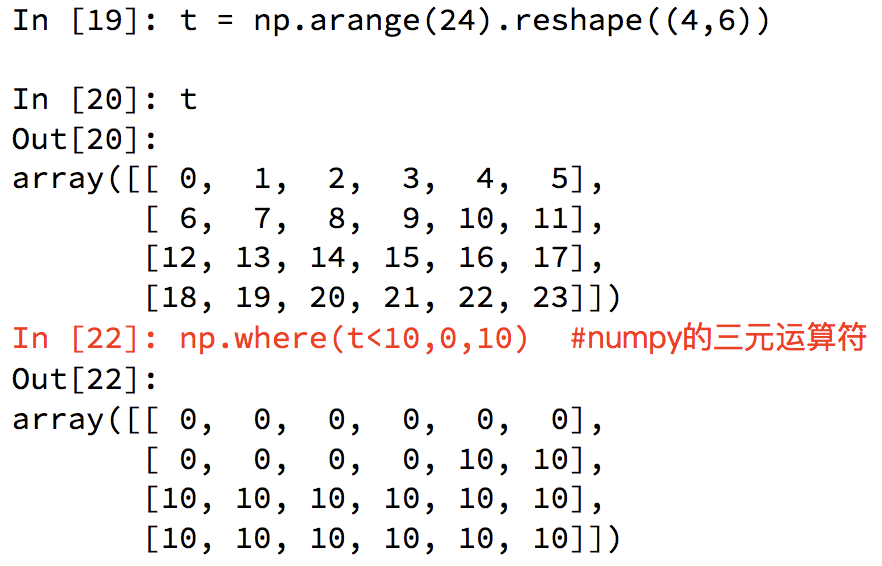numpy中的clip(裁剪)

• 小于10的替换为10，大于18的替换为了18，但是nan没有被替换。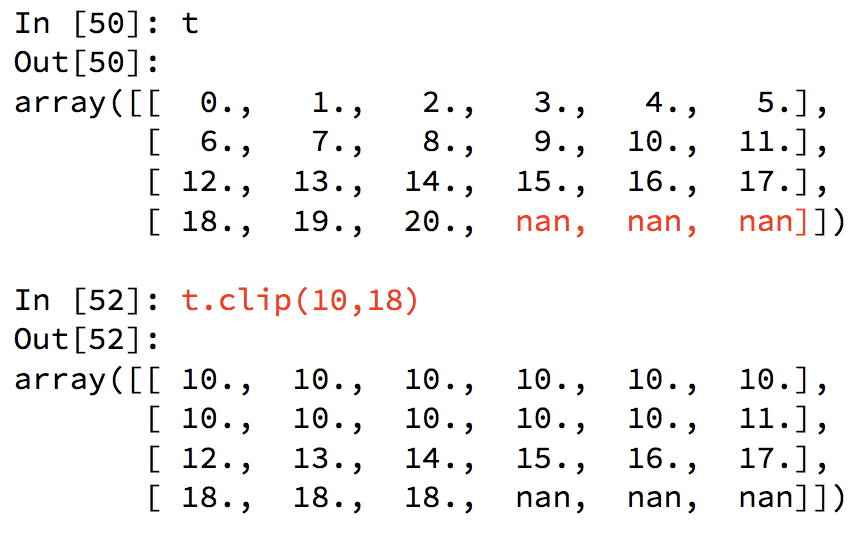numpy中的nan和inf

• Nan(Nan,Nan)：表示不是一个数字
• np.nan，type=float
• 什么时候出现nan：
• 当我们读取本地的文件为float的时候，如果有缺失，就会出现nan。
• 当做了一个不合适的计算的时候，比如无穷大(inf)减去无穷大。
• inf(-inf,inf)：inf表示正无穷，-inf表示负无穷
• np.inf，type=float
• 什么时候出现inf：
• 比如一个数字除以0，python中直接会报错，numpy中是一个inf或者-inf。

numpy中的nan的注意点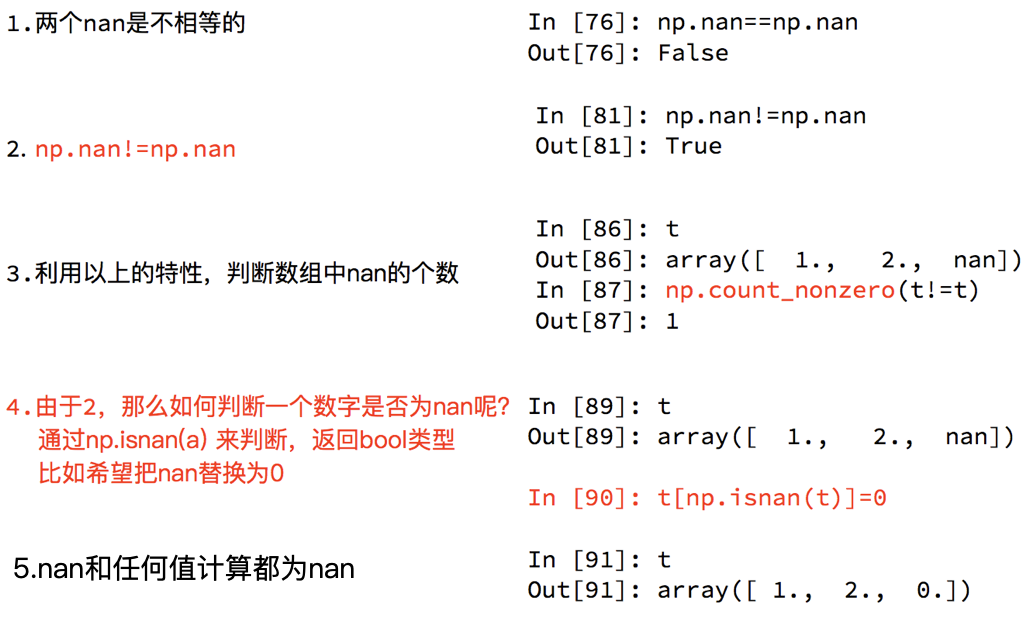• 统计nan的个数
• np.count_nonzero(t2!=t2)
• np.count_nonzero(np.isnan(t2))
• nan和任何值计算都为nan
• 若t2中有nan，则np.sum(t2)返回nan
• 因此在进行计算的时候，希望吧nan进行赋值来计算，但不能把他们全部替换为0
• 全部替换为0后，替换之前的平均值如果大于0，替换之后的均值肯定会变小，所以一般
• 把缺失的数值替换为均值（中值）
• 直接删除有缺失值的一行。

ndarry缺失值填充均值

# coding=utf-8
import numpy as np

def fill_ndarray(t1):
# 遍历每一列
for i in range(t1.shape)：
temp_col = t1[:, i]	# 当前的一列
nan_num = np.count_nonzero(temp_col!=temp_col)	#  找出值为nan的元素的个数
if nan_num != 0:	# 不为0，说明当前这一列中有nan
temp_not_nan_col = temp_col[temp_col==temp_col]	# 当前一列不为nan的array
temp_not_nan_col.mean()
# 选中当前为nan的位置，赋值为不为nan的，本列的均值
temp_col[np.isnan(temp_col)] = temp_not_nan_col.mean()
return t1

if __name__ == "__main__":
t1 = np.arrange(12).reshape((3,4)).astype("float")
t1[1, 2:] = np.nan	# 设置2行3、4列的值为nan
t1 = fill_ndarray(t1)数组的拼接

• 竖直分割与竖直拼接互为inverse。
• 竖直拼接（vertically）：np.vstack((t1, t2))
• 注意：需要确定每一列代表的意义相同！！！否则牛头不对马嘴。
• 水平拼接（horizontally）：np.hstack((t1, t2))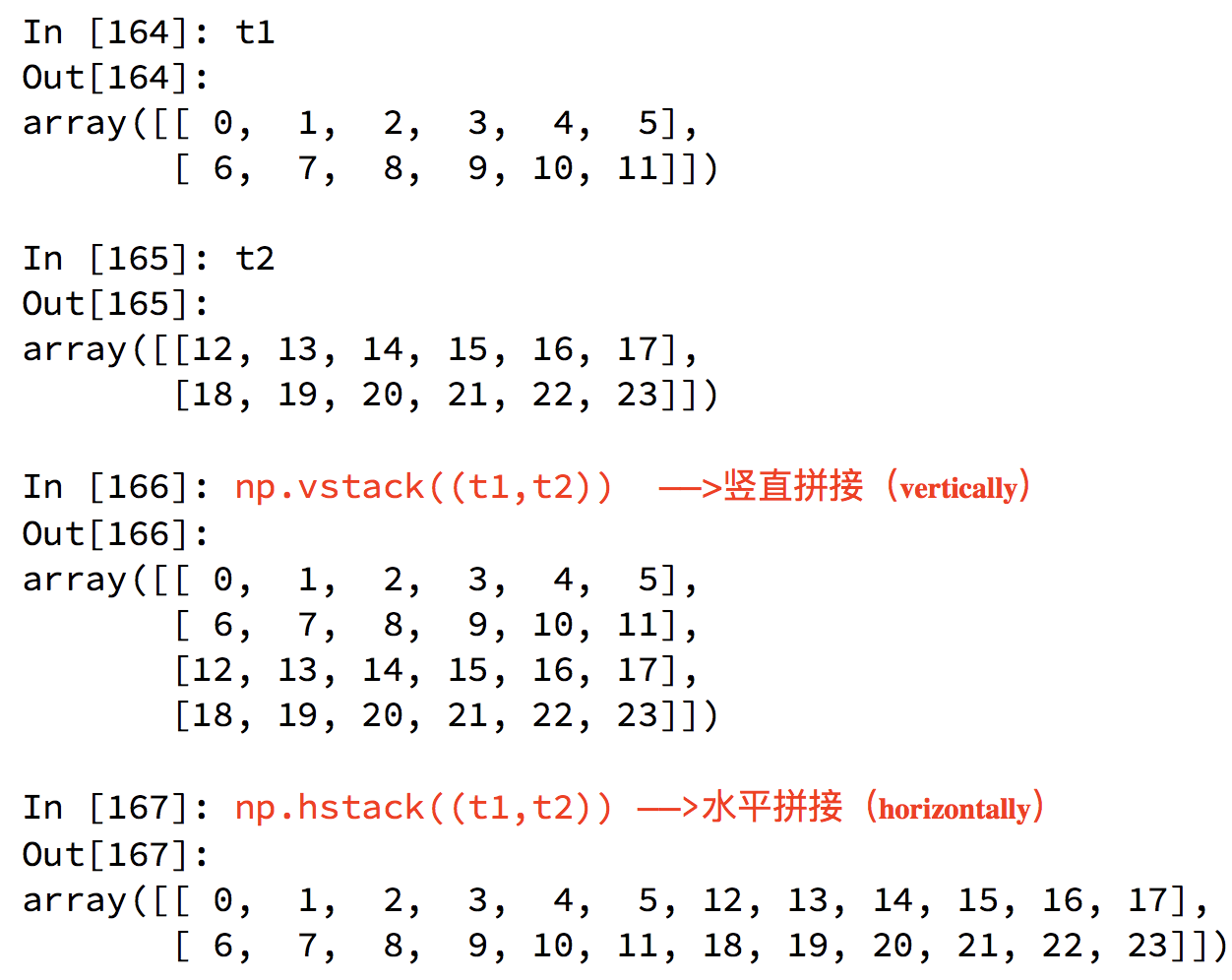数组的行列交换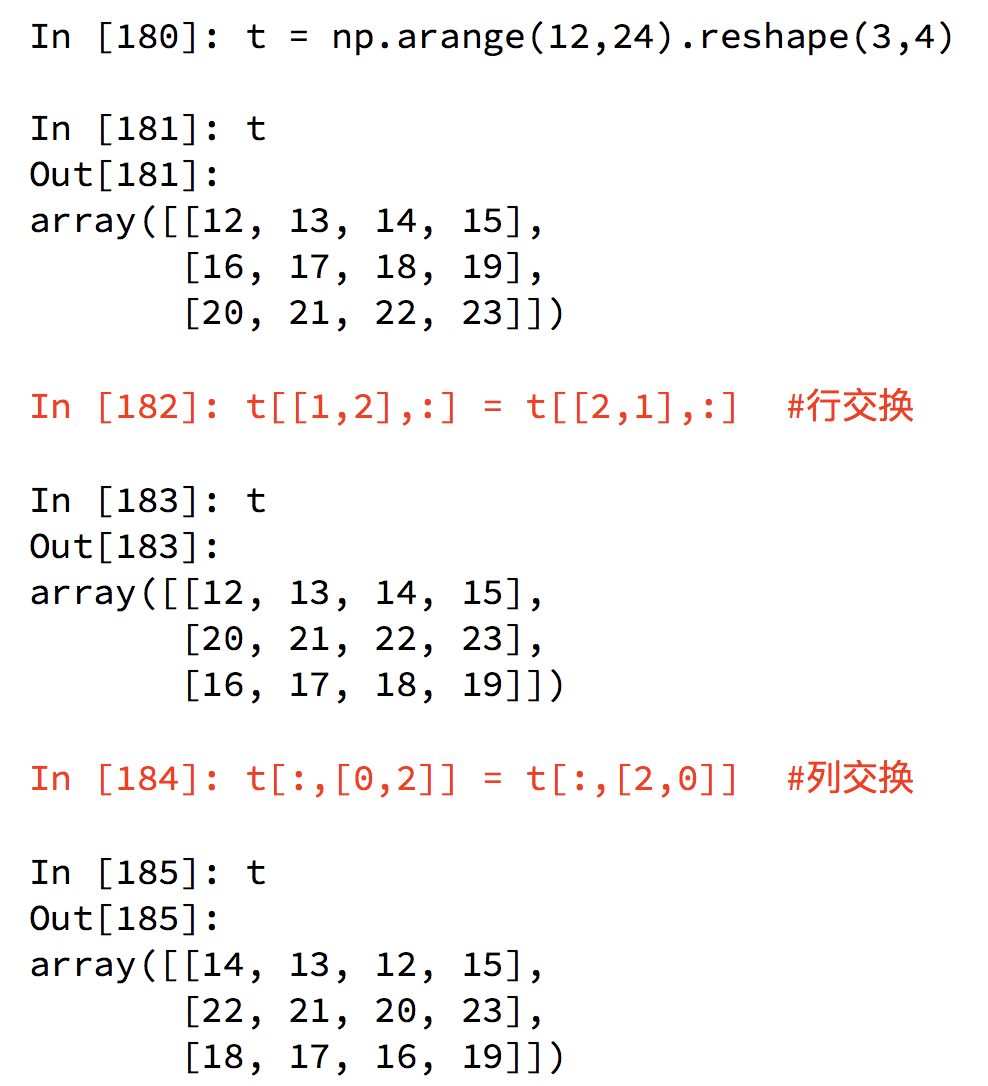数组的拼接和行列交换的实现

• 现在希望把之前案例中两个国家的数据方法一起来研究分析，同时保留国家的信息（每条数据的国家来源），应该怎么办？
# coding=utf-8
import numpy as np

# 加载国家数据

# 添加国家信息
# 构造全为0的列：us_data.shape获得列表行数
zeros_data = np.zeros((us_data.shape, 1)).astype(int)
# 构造全为1的列
ones_data = np.ones((uk_data.shape, 1)).astype(int)

# 分别添加一列全为0的数据和全为1的数据
us.data = np.hstack((us_data, zeros_data))
uk.data = np.hstack((uk_data, ones_data))

# 拼接两组数据
final_data = np.vstack((us_data, uk_data))numpy更多好用的方法

• 获取最大值最小值的位置
• 每一列的最大值：np.argmax(t, axis=0)，和行的形状是一样的
• 每一行的最大值：np.argmin(t, axis=1)，和列的形状是一样的
• 创建一个全0的数组：np.zeros((3,4))
• 创建一个全1的数组：np.ones((3,4))
• 创建一个

3

3

3*3

的identity matrix：np.eye(3)

numpy生成随机数

• np.random.—-
• 生成一个3×4的数组，每个元素0～19内随机生成：np.random.randint(0, 20, (3, 4))
• 固定随机数，随机数按着固定的顺序出现：np.random.seed(5)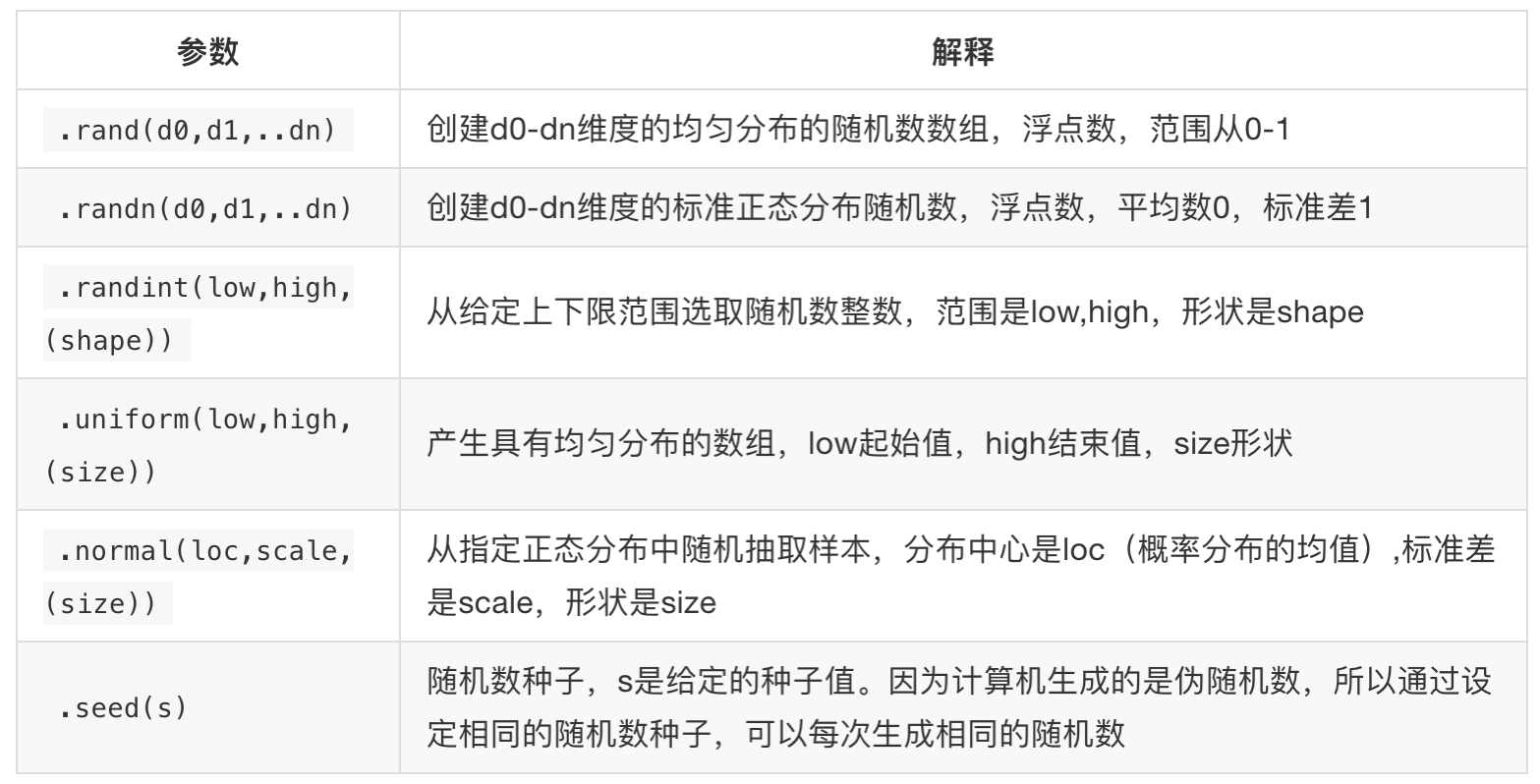numpy常用统计方法

• 默认返回多维数组的全部的统计结果,如果指定axis则返回一个当前轴上的结果
• 在每一列上操作：axis=0，和行的形状是一样的
• 在每一行上操作：axis=1，和列的形状是一样的
• 求和：t.sum(axis=None)
• 均值：t.mean(a,axis=None) 受离群点的影响较大
• 中值：np.median(t,axis=None)
• 最大值：t.max(axis=None)
• 最小值：t.min(axis=None)
• 极值：np.ptp(t,axis=None) 即最大值和最小值只差
• 标准差：t.std(axis=None) 越大波动越大

numpy和matplotlib的一个具体应用

# coding=utf-8
import numpy as np
from matplotlib import pyplot as plt

# 取评论的数据

# 选择比5000小的数据

d = 250
bin_mums = (t_us_comments.max() - t_us_comments.max()) // d	# max = 500000, min = 0, 极差过大，但大多数值差异没有那么大，所以需要去掉过大的值。如果bin_nums除不尽的话，图像会发生偏移

# 绘图
plt.figure(figuresize=(20, 8), dpi=80)

plt.show()# 选择比500000小的数据（以此来凸出过小的变化们）
t_us = t_us[t_us[:,1]<=500000]

t_us_comment = t_us[:, -1]
t_us_like = t_us[:, 1]

plt.figure(figuresize=(20, 8), dpi=80)
plt.scatter(t_us_like, t_us_comment)

plt.show()总结评论 抢沙发觉得文章有用就打赏一下文章作者

支付宝扫一扫打赏微信扫一扫打赏Vieu3.3主题Q Q 登 录